## Tuesday, November 21, 2017

### Fun with indicator constraints

In  a simple model is presented for the Least Median of Squares regression problem:

 \begin{align}\min\>&z\\&\delta_i=1 \Rightarrow \> –z \le r_i \le z\\&\sum_i \delta_i = h\\&r_i = y_i - \sum_j X_{i,j} \beta_j\\&\delta_i \in \{0,1\} \end{align}

Here $$X$$ and $$y$$ are data. Modern solvers like Cplex and Gurobi support indicator constraints. This allows us to directly pass on the above model to a solver without resorting to other formulations (such as binary variables with big-M constraints or SOS1 structures).

This particular model is interesting: it has an unbounded LP relaxation. E.g. the start of the Cplex log shows:

 Nodes                                         Cuts/    Node  Left     Objective  IInf  Best Integer    Best Bound    ItCnt     Gap       0     0     unbounded                                          0               0     2     unbounded                                          0         Elapsed time = 0.03 sec. (1.27 ticks, tree = 0.01 MB, solutions = 0) *    44    44      integral     0        0.4886       -0.0000       63  100.00% Found incumbent of value 0.488611 after 0.05 sec. (3.02 ticks) *    45    43      integral     0        0.4779       -0.0000       66  100.00%. . .

Gurobi has some real problems on this model:

 Optimize a model with 48 rows, 97 columns and 188 nonzeros Model has 94 general constraints Variable types: 50 continuous, 47 integer (47 binary) Coefficient statistics:   Matrix range     [1e+00, 5e+00]   Objective range  [1e+00, 1e+00]   Bounds range     [1e+00, 1e+00]   RHS range        [4e+00, 2e+01] Presolve added 47 rows and 47 columns Presolve time: 0.00s Presolved: 95 rows, 144 columns, 423 nonzeros Presolved model has 94 SOS constraint(s) Variable types: 97 continuous, 47 integer (47 binary) Root relaxation: unbounded, 77 iterations, 0.00 seconds     Nodes    |    Current Node    |     Objective Bounds      |     Work Expl Unexpl |  Obj  Depth IntInf | Incumbent    BestBd   Gap | It/Node Time      0     0  postponed    0               -          -      -     -    0s     0     0  postponed    0               -          -      -     -    0s     0     2  postponed    0               -          -      -     -    0s *  136   136              68       0.9658407          -      -   6.1    0s *  138   136              69       0.9135526          -      -   6.4    0s H  363   353                       0.8212338          -      -   5.1    0s H  997   786                       0.6097619          -      -   5.5    0s H 1414   964                       0.5006481          -      -   6.9    0s H 1564   892                       0.3806481          -      -   8.7    0s H 1713   931                       0.3611765          -      -   8.8    0s...139309129 6259738    0.02538   65   23    0.26206          -      -  28.3 28775s 139337799 6262622     cutoff   67         0.26206          -      -  28.3 28780s 139367156 6266141     cutoff   68         0.26206          -      -  28.3 28785s 139395590 6268935     cutoff   67         0.26206          -      -  28.3 28790s 139424342 6272187  postponed   64         0.26206          -      -  28.3 28795s

This model does not seem to finish (the model has only 47 discrete variables so this log indicates something is seriously wrong: this model should solve in a manner of seconds). Gurobi was never able to establish a lower bound (column BestBd). Notice that Gurobi translates the implications to SOS1 variables (see  for the SOS1 version of this model).

There is a simple work around: add the bound $$z \ge 0$$. Now things solve very fast. We have a normal bound and no more any of these “postponed” nodes.

 Root relaxation: objective 0.000000e+00, 52 iterations, 0.00 seconds     Nodes    |    Current Node    |     Objective Bounds      |     Work Expl Unexpl |  Obj  Depth IntInf | Incumbent    BestBd   Gap | It/Node Time      0     0    0.00000    0   24          -    0.00000      -     -    0s H    0     0                       0.7556195    0.00000   100%     -    0s     0     2    0.00000    0   24    0.75562    0.00000   100%     -    0s H   54    30                       0.5603846    0.00000   100%  19.3    0s. . .

The bound $$z\ge 0$$ can be deduced from the constraints $$–z \le r_i \le z$$ (we know $$h$$ of them have to hold). Presolvers are not able to find this out automatically.

See  for some other interesting observations on indicator constraints.

Update: Gurobi has identified the problem and it has been fixed (available in next version).

##### References
1. Integer Programming and Least Median of Squares Regression, http://yetanothermathprogrammingconsultant.blogspot.com/2017/11/integer-programming-and-least-median-of.html
2. Belotti, P., Bonami, P., Fischetti, M. et al., On handling indicator constraints in mixed integer programming, Comput Optim Appl (2016) 65: 545.

## Tuesday, November 14, 2017

### Integer Programming and Least Median of Squares Regression

Least Median Regression  is another attempt to make regression more robust, i.e. less sensitive to outliers.

 \begin{align}\min_{\beta}\>&\operatorname*{median}_i r_i^2 \\ &y-X\beta = r\end{align}

From the summary of :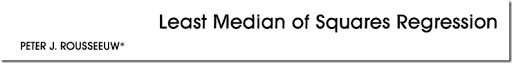Classical least squares regression consists of minimizing the sum of the squared residuals. Many authors have produced more robust versions of this estimator by replacing the square by something else, such as the absolute value. In this article a different approach is introduced in which the sum is replaced by the median of the squared residuals. The resulting estimator can resist the effect of nearly 50% of contamination in the data. In the special case of simple regression, it corresponds to finding the narrowest strip covering half of the observations. Generalizations are possible to multivariate location, orthogonal regression, and hypothesis testing in linear models.

For $$n$$ even, the median is often defined as the average of the two middle observations. For this regression model, usually a slightly simplifying definition is used: the median is the $$h$$-th ordered residual with $$h=\lfloor (n+1)/2\rfloor$$ (here $$\lfloor . \rfloor$$ means rounding down). For technical reasons, sometimes another value is suggested :

 $h=\left\lfloor \frac{n}{2} \right\rfloor + \left\lfloor \frac{p+1}{2}\right\rfloor$

where $$p$$ is the number of coefficients to estimate (number of $$\beta_j$$’s). Some algorithms use $$\lfloor (n+p+1)/2\rfloor$$. The objective function can therefore be described succinctly as:

 $\min\>r^2_{(h)}$

We used here the ordering $$|r|_{(1)}\le |r|_{(2)}\le \dots\le|r|_{(n)}$$. The problem for general $$h$$’s is called least quantile of squares regression or shorter least quantile regression. For $$h=n$$ the model can be reformulated as a Chebyshev regression problem .

##### Intermezzo: Minimizing k-th smallest

Minimizing the $$k$$-th smallest value $$x_{(k)}$$ of a set of variables $$x_i$$, with $$i=1,\dots n$$ is not obvious. Minimizing the largest value, $$x_{(n)}$$ is easy: we can do

 \begin{align}\min\>&z\\&z\ge x_i\end{align}

The trick to minimize $$x_{(k)}$$ is to do:

 \begin{align}\min\>&z\\&z\ge x_i- M \delta_i\\&\sum_i\delta_i = n-k\\&\delta_i \in \{0,1\}\end{align}

In effect we dropped the largest $$n-k$$ values from consideration (in statistical terms this is called “trimming”).  To be precise: we dropped $$n-k$$ values, and because we are minimizing, these will be the largest values automatically. Note that we do not sort the values here: sorting values in a MIP model is very expensive.

The LMS model can now be stated as:

 \begin{align}\min\>&z\\&z\ge r^2_i-M\delta_i\\ &\sum_i \delta_i = n-h\\&r_i = y_i-\sum_j X_{i,j} \beta_j\\&\delta_i \in \{0,1\}\end{align}

This is a MIQCP (Mixed Integer Quadratically Constrained Programming) model. It is convex so we can solve this with solvers like Cplex and Gurobi.

##### An MINLP model

Redefining our $$\delta_i$$ variables, we can formulate a MINLP model:

 \begin{align}\min\>&z\\&z\ge \delta_i \cdot r^2_i\\ &\sum_i \delta_i = h\\&r_i = y_i-\sum_j X_{i,j} \beta_j\\&\delta_i \in \{0,1\}\end{align}

This model is very compact but requires an MINLP solver. Finding globally optimal solutions for this problem is not very easy.

##### Absolute values

Minimizing the median of the squared errors is the same as minimizing the median of the absolute values of the errors. This is because the ordering of squared errors is the same as the ordering of the absolute values. Hence the objective of our problem can be stated as:

 $\min\>|r|_{(h)}$

i.e., minimize the $$h$$-th smallest absolute value.

Now we know how to minimize the $$h$$-th smallest value, the only hurdle left is combining this with an absolute value formulation. We follow here the sparse bounding formulation from [3,4].

 \begin{align}\min\>&z\\&-z - M\delta_i \le r_i \le z + M\delta_i\\&\sum_i \delta_i = n-h\\&r_i = y_i-\sum_j X_{i,j} \beta_j\\&\delta_i \in \{0,1\}\end{align}
It is not so easy to find good values for these big-$$M$$’s (see  for an attempt). One way around this is to use indicator constraints:
 \begin{align}\min\>&z\\&\delta_i=1 \Rightarrow \> –z \le r_i \le z\\&\sum_i \delta_i = h\\&r_i = y_i-\sum_j X_{i,j} \beta_j\\&\delta_i \in \{0,1\} \end{align}

Note that we have flipped the meaning of $$\delta_i$$. If your solver does not allow for indicator constraints, we can also achieve the same thing by SOS1 sets:

 \begin{align}\min\>&z\\&-z-s_i\le r_i \le z + s_i\\&s_i\cdot \delta_i = 0\\&\sum_i \delta_i = h\\&r_i = y_i-\sum_j X_{i,j} \beta_j\\&\delta_i \in \{0,1\}\end{align}

where $$s_i$$ are additional slack variables. They can be free or non-negative variables. The complementarity condition $$s_i\cdot \delta_i = 0$$ can be implemented by forming $$n$$ SOS1 sets with two members: $$(\delta_i,s_i)$$. Actually some solvers may reformulate indicator constraints into SOS1 sets when there are no good bounds (in which case a direct translation to a big-M binary variable reformulation is not possible).

A similar but more complicated approach using variable splitting has been proposed by :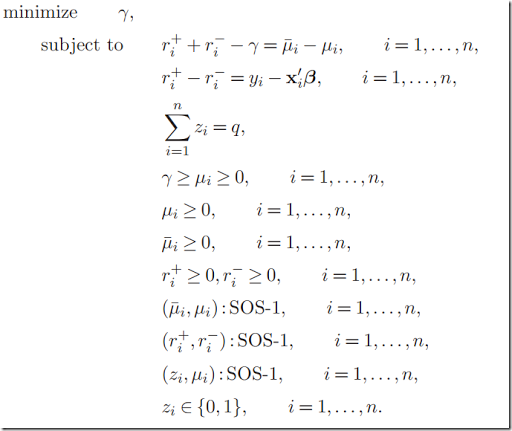Besides being unnecessarily complex, I believe this formulation is not correct. An obvious optimal solution for this model would be $$\gamma=0$$ and $$\mu_i=0$$ which is not a reasonable solution for our original problem. One way to repair this model is by saying the pairs $$(z_i,\bar{\mu}_i)$$ form SOS1 sets instead of $$(z_i,\mu_i)$$. I assume this is what the authors meant. This change makes the model correct, but not any simpler.

If we really want to use a variable splitting approach with SOS1 sets, I propose a much simpler model:

 \begin{align}\min\>&z\\&z \ge r_i^+ + r_i^- - s_i\\&r_i^+ - r_i^- = y_i – \sum_j X_{i,j}\beta_j\\&s_i\cdot \delta_i = 0\\&\sum_i \delta_i = h\\&\delta_i \in \{0,1\}\\&r_i^+, r_i^-, s_i \ge 0\end{align}

The variable splitting in this model is not immediately interpretable: we only guarantee $$|r_i|=r_i^+ + r_i^-$$ for the case where $$s_i=0$$ and $$z \ge r_i^+ + r_i^-$$ is binding.

##### Example

In [6,7] a dramatic example is shown on a small data set.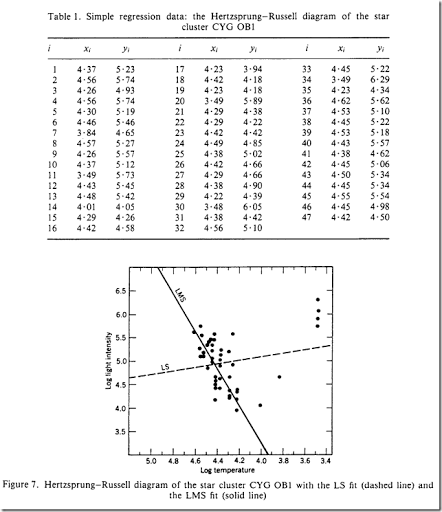Another interesting plot is to compare the ordered absolute values of the residuals: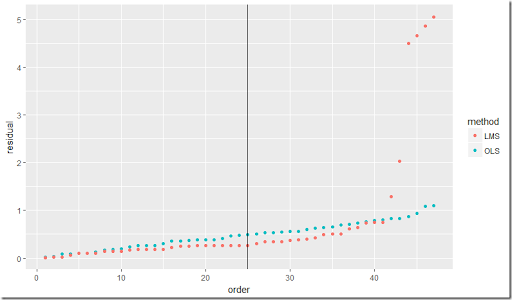Here we plotted $$|r|_{(i)}$$. The model was solved with $$h=25$$ (this is where the vertical line is drawn). As you can see, LMS (Least Median of Squares) tries to keep the absolute value of the $$h$$-th residual as small as possible, and does not care about what happens afterward. We can see the residual increase in magnitude after this point. OLS (Ordinary Least Squares) will try to minimize all squared residuals and thus is heavily influenced by the bigger ones at the end.

##### Some timings

The “k out of n” constraint is always difficult for MIP solvers. We see that also here using Cplex: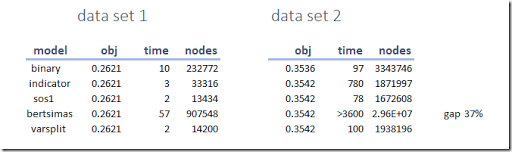Our fixed version of the model from  is doing somewhat poorly: it has too many discrete structures. (In  a really good approximation is found and then passed to the MIP using MIPSTART).

Data set 2 has a few interesting results. The binary variable approach with big-M’s leads to a better than optimal solution due to “trickle flow”. One of the binary variables has value: 3.5193967E-6 which is within the integer feasibility tolerance but is large enough to cause the objective to improve a bit. The Bertsimas model found the optimal solution within an hour but we were not able to prove global optimality (it still has a large relative gap).

##### A note on minimizing the true median

Going back to the standard definition of the median, the “true” median is usually defined as the average of the two middle observations in case $$n$$ is even. In the above discussion we use a simpler definition and just minimized the $$h$$-th ordered residual. Here I’ll try to show that it is possible to handle the traditional definition of the median. This requires a little bit of effort however.

The trick to minimize the median in general can look like :

If $$n$$ is odd:

 \begin{align}\min\>&z\\&z\ge x_i-\delta_i M\\&\sum_i \delta_i = \frac{n-1}{2}\\&\delta_i \in \{0,1\}\end{align}

If $$n$$ is even:

 \begin{align}\min\>&\frac{z_1+z_2}{2}\\&z_1\ge x_i-\delta_i M\\&\sum_i \delta_i = \frac{n}{2}\\&z_2\ge x_i-\eta_i M\\&\sum_i \eta_i = \frac{n}{2}-1\\&\delta_i,\eta_i \in \{0,1\}\end{align}

The “$$n$$ is even” case is the new and interesting part. Note that I expect we can improve this “$$n$$ is even” version a little bit by adding the condition $$\delta_i \ge \eta_i$$.

This technique allows us to calculate the minimum median of a variable $$x_i$$. However, if we want to apply this to our Least Median of Squares model we are in big trouble. In our formulations, we used a trick that minimizing $$r^2_{(h)}$$ is the same as minimizing $$|r|_{(h)}$$. This is no longer the case with this “true” median objective for $$n$$ even. We can however use a quadratic formulation:

If $$n$$ is even:

 \begin{align}\min\>&\frac{z_1+z_2}{2}\\&z_1\ge r^2_i-\delta_i M\\&\sum_i \delta_i = \frac{n}{2}\\&z_2\ge r^2_i-\eta_i M\\&\sum_i \eta_i = \frac{n}{2}-1\\&r_i = y_i-\sum_j X_{i,j} \beta_j\\&\delta_i,\eta_i \in \{0,1\}\end{align}

Although we can solve this with standard solvers, it turns out to be quite difficult. The linear models discussed before were much easier to solve.

This regression problem turns out to be interesting from a modeling perspective.

##### References
1. Peter Rousseeuw, Least Median of Squares Regression, Journal of the American Statistical Association, 79 (1984), pp. 871-880.
2. A. Giloni, M. Padberg, Least Trimmed Squares Regression, Least Median Squares Regression, and Mathematical Programming, Mathematical and Computer Modelling 35 (2002), pp. 1043-1060
4. Linear programming and Chebyshev regression, http://yetanothermathprogrammingconsultant.blogspot.com/2017/11/lp-and-chebyshev-regression.html
5. Dimitris Bertsimas and Rahul Mazumder, Least Quantile Regression via Modern Optimization, The Annals of Statistics, 2014, Vol. 42, No. 6, 2494-2525, https://arxiv.org/pdf/1310.8625.pdf.
6. Peter Rousseeuw, Annick Leroy, Robust Regression and Outlier Detection, Wiley, 2003.
7. Peter Rousseeuw, Tutorial to Robust Statistics, Journal of Chemometrics, Vol. 5, pp. 1-20, 1991.
8. Paul Rubin, Minimizing a Median, https://orinanobworld.blogspot.com/2017/09/minimizing-median.html

## Friday, November 10, 2017

### Linear Programming and Chebyshev Regression

The LAD (Least Absolute Deviation) or $$\ell_1$$ regression problem (minimize the sum of the absolute values of the residuals) is often discussed in Linear Programming textbooks: it has a few interesting LP formulations . Chebyshev or $$\ell_{\infty}$$ regression is a little bit less well-known. Here we minimize the maximum (absolute) residual.

\bbox[lightcyan,10px,border:3px solid darkblue] {\begin{align}\min_{\beta}\>&\max_i \> |r_i|\\ &y-X\beta = r\end{align}}

As in ,  $$\beta$$ are coefficients to estimate and  $$X,y$$ are data. $$r$$ are the residuals.
Some obvious and less obvious formulations are:
• Variable splitting:\begin{align}\min\>&z\\& z \ge r^+_i + r^-_i\\&r^+_i - r^-_i =y_i –\sum_j X_{i,j}\beta_j\\&r^+_i, r^-_i\ge 0\end{align} With variable splitting we use two non-negative variables $$r^+_i - r^-_i$$ to describe a value $$r_i$$ that can be positive or negative. We need to make sure that one of them is zero in order for $$r^+_i + r^-_i$$ to be equal to the absolute value $$|r_i|$$. Here we have an interesting case, as we are only sure of this for the particular index $$i$$ that has the largest absolute deviation (i.e. where $$|r_i|=z$$). In cases where $$|r_i|<z$$ we actually do not  enforce $$r^+_i \cdot r^-_i = 0$$. Indeed, when looking at the solution I see lots of cases where $$r^+_i > 0, r^-_i > 0$$. Effectively those $$r^+_i, r^-_i$$ have no clear physical interpretation. This is very different from the LAD regression formulation  where we require all $$r^+_i \cdot r^-_i = 0$$.
• Bounding:\begin{align}\min\>&z\\ & –z \le y_i –\sum_j X_{i,j}\beta_j \le z\end{align}Here $$z$$ can be left free or you can make it a non-negative variable (it will be non-negative automatically). Note that there are actually two constraints here: $$–z \le y_i –\sum_j X_{i,j}\beta_j$$ and $$y_i –\sum_j X_{i,j}\beta_j \le z$$. This model contains the data twice, leading to a large number of nonzero elements in the LP matrix.
• Sparse bounding: This model tries to remedy the disadvantage of the standard bounding model by introducing extra free variables $$d$$ and extra equations:\begin{align}\min\>&z\\ & –z \le d_i \le z\\& d_i = y_i –\sum_j X_{i,j}\beta_j \end{align} Note again that $$–z \le d_i \le z$$ is actually two constraints: $$–z \le d_i$$ and $$d_i \le z$$.
• Dual:\begin{align}\max\>&\sum_i y_i(d_i+e_i)\\&\sum_i X_{i,j}(d_i+e_i) = 0 \perp \beta_j\\&\sum_i (d_i-e_i)=1\\&d_i\ge 0, e_i\le 0\end{align}The estimates $$\beta$$ can be found to be the duals of equation $$X^T(d+e)=0$$.
We use the same synthetic data as in  with $$m=5,000$$ cases, and $$n=100$$ coefficients. Some timings with Cplex (default LP method) yield the following results (times are in seconds):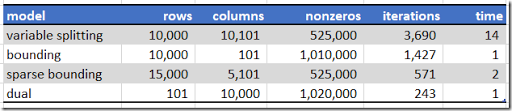Opposed to the LAD regression example in , the bounding formulation is very fast here. The dual formulation remains doing very good.
##### Historical Note
The use of this minimax principle goes back to Euler (1749) [3,4].Leonhard Euler (1707-1783)
The term Chebyshev regression is related to the Chebyshev distance (a different name for the $$\ell_{\infty}$$ metric).Pafnuty Lvovich Chebyshev (1821-1894)

##### References
2. A.Giloni, M.Padberg, Alternative Methods of Linear Regression, Mathematical and Computer Modeling 35 (2002), pp.361-374.
3. H.L. Harter, The method of least squares and some alternatives, Technical Report, Aerospace Research Laboratories, 1972.
4. L. Euler, Pièce qui a Remporté le Prix de l'Academie Royale des Sciences en 1748, sur les Inegalités de Mouvement de Saturn et de Jupiter. Paris (1749).

## Thursday, November 9, 2017

### Linear Programming and LAD Regression

I believe any book on linear programming will mention LAD (Least Absolute Deviation) or $$\ell_1$$ regression: minimize the sum of the absolute values of the residuals.

 \begin{align}\min_{\beta}\>&\sum_i |r_i|\\ &y-X\beta = r\end{align}

Here $$\beta$$ are coefficients to estimate. So they are the decision variables in the optimization model. $$X,y$$ are data (watch out here: in many optimization models we denote decision variables by $$x$$; here they are constants). $$r$$ are the residuals; it is an auxiliary variable that can be substituted out.

The standard LP models suggested for this problem are not very complicated, but still interesting enough to have them cataloged.

There are at least three common LP formulations for this: variable splitting, bounding and a dual formulation. Here is a summary:

• Variable splitting:\begin{align}\min\>&\sum_i r^+_i + r^-_i\\&r^+_i - r^-_i =y_i –\sum_j X_{i,j}\beta_j\\&r^+_i, r^-_i\ge 0\end{align}In this model, automatically one of the pair $$(r^+_i,r^-_i)$$ will be zero. We don’t have to add an explicit complementarity condition $$r^+_i \cdot r^-_i = 0$$. This is fortunate: we can keep the model linear.
• Bounding:\begin{align}\min\>&\sum_i r_i\\&-r_i \le y_i –\sum_j X_{i,j}\beta_j \le r_i\end{align}Here $$r_i$$ can be left free or you can make it a non-negative variable. It will be non-negative automatically. Note that there are actually two constraints here: $$-r_i \le y_i –\sum_j X_{i,j}\beta_j$$ and $$y_i –\sum_j X_{i,j}\beta_j\le r_i$$. This formulation is mentioned in .
• Sparse bounding:
In the standard bounding formulation we have all the data $$X_{i,j}$$ twice in the model, leading to a large number of non-zero elements in the LP matrix. We can alleviate this by introducing an extra free variable $$d$$: \begin{align}\min\>&\sum_i r_i\\&-r_i \le d_i \le r_i\\&d_i = y_i –\sum_j X_{i,j}\beta_j\end{align} Effectively we reduced the number of non-zeros by a factor of two compared to the standard bounding formulation. Note that the first constraint $$-r_i\le d_i \le r_i$$ is actually two constraints: $$-r_i\le d_i$$ and $$d_i\le r_i$$. The sparse version will have fewer non-zero elements, but many more constraints and variables. Advanced LP solvers are based on sparse matrix technology and the effect of more nonzeros is often underestimated by novice users of LP software. The same arguments and reformulations can be used in $$\ell_1$$ portfolio models .
• Dual:\begin{align}\max\>&\sum_i y_i d_i\\&\sum_i X_{i,j} d_i=0 \perp \beta_j \\&-1\le d_i \le 1\end{align} The optimal values for $$\beta$$ can be recovered from the duals for the constraint $$X^Td=0$$ (this is what the notation $$\perp$$ means).

One could make the argument the last formulation is the best: it has fewer variables than variable splitting, and fewer equations than the bounding approach. In addition, as mentioned before, the standard bounding formulation has the data twice in the model, resulting in a model with many nonzero elements.

Modern LP solvers are not very well suited for these type of models. They like very sparse LP models, while these models are very dense. Let’s try anyway with a large, artificial data set with $$m=5,000$$ cases, and $$n=100$$ coefficients. The data matrix $$X$$ has 500,000 elements. Some timings with Cplex (default LP method) yield the following results: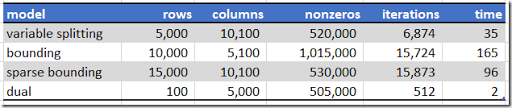Times are in seconds.

The dual formulation seems indeed quite fast. It is interesting that the bounding model (this formulation is used a lot) is actually the slowest. Note that these results were obtained using default settings. This effectively means that Cplex selected the dual simplex solver for all these instances. These timings will change when the primal simplex method or the barrier algorithm is used.

##### L1fit

The R package L1pack  contains a dense, simple (compared to say Cplex), specialized version of the Simplex method based on an algorithm from [6,7]. It is actually quite fast on the above data: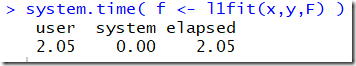The number of Simplex iterations is 561. This confirms that sparse, general purpose LP solvers have a big disadvantage on this problem (usually Cplex would beat any LP algorithm you can come up yourself, by a large margin).

See the comment from Robert Fourer for some notes on the Barrodale-Roberts algorithm. So I think I need to refine my previous statement a bit: there are two reasons why l1fit is doing so well: (1) dense data using a dense code and (2) a specialized Simplex method handling the absolute values. The number of iterations is close to our dual formulation (which is also very compact), so the time per iteration is about the same as Cplex.

##### Historical note

The concept of minimizing the sum of absolute deviations goes back to .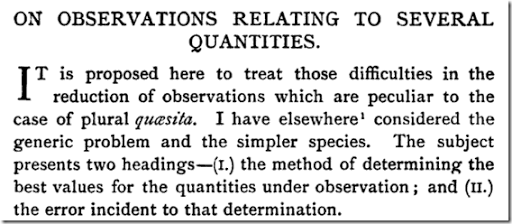The term quaesita refers to “unknown quantities”.F.Y. Edgeworth, 1845-1926.

I noticed that Edgeworth was from Edgeworthstown, Ireland (population: 2,335 in 2016).##### References
1. Least absolute deviations, https://en.wikipedia.org/wiki/Least_absolute_deviations
2. A.Giloni, M.Padberg, Alternative Methods of Linear Regression, Mathematical and Computer Modeling 35 (2002), pp.361-374.
3. L1 portfolio formulation, http://yetanothermathprogrammingconsultant.blogspot.com/2010/04/l1-portfolio-formulation.html
4. Francis Ysidro Edgeworth, On observations relating to several quantities, Hermathena 6 (1887), pp. 279-285
5. Osorio, F., and Wołodźko, T. (2017). L1pack: Routines for L1 estimation, https://cran.r-project.org/web/packages/L1pack/index.html
6. Barrodale, I., and Roberts, F.D.K. (1973). An improved algorithm for discrete L1 linear approximations. SIAM Journal of Numerical Analysis 10, 839-848
7. Barrodale, I., and Roberts, F.D.K. (1974). Solution of an overdetermined system of equations in the L1 norm. Communications of the ACM 17, 319-320.

## Tuesday, November 7, 2017

### Suguru: GAMS/MIP vs Python/Z3

 For many logical puzzles a constraint solver like Z3 may be more suitable than a Mathematical Programming approach using a MIP solver. In  a MIP formulation was presented for Suguru puzzle. Now let’s see how much difference we observe when we use Z3.

There are two major implications for the model itself:

1. We can use integers directly, i.e.  $$x_{i,j} \in \{1,\dots,u_{i,j}\}$$ instead of $$x_{i,j,k} \in \{0,1\}$$.

However most of the code is devoted to data manipulation as we will see below. Although the approaches are different (different languages, different solver technologies), the actual code is remarkably similar.

##### Data entry: GAMS

First we need to get the data into GAMS. Unfortunately we need to enter two matrices: one for the blocks and one for the initial values.

 set  i 'rows' /i1*i7/  j 'columns' /j1*j9/  k 'values' /1*9/  b 'blocks' /b1*b15/;table blockno(i,j)      j1 j2 j3 j4 j5 j6 j7 j8 j9  i1   1  1  2  2  2  3  3  4  6  i2   1  1  2  2  3  3  4  4  6  i3   1  7  8  8  3  4  4  5  6  i4   7  7  7  8  8  9  9  6  6  i5   7 10 10  8 12  9  9 14 15  i6  11 11 10 10 12 12  9 15 15  i7  11 11 10 12 12 13 13 15 15;table initval(i,j)      j1 j2 j3 j4 j5 j6 j7 j8 j9  i1   1        1  i2      2                 3  i3   4     4              1  4  i4            1  i5   4     5     4     5  1  i6                        3  i7               2           5;

The set k is a bit of an over-estimate: we actually need only values 1 through 5 for this problem (the maximum size of a block is 5).

##### Data entry: Python

Entering the same boards in Python can look like:

 from z3 import * blockno = [ [ 1, 1, 2, 2, 2, 3, 3, 4, 6],             [ 1, 1, 2, 2, 3, 3, 4, 4, 6],             [ 1, 7, 8, 8, 3, 4, 4, 5, 6],             [ 7, 7, 7, 8, 8, 9, 9, 6, 6],             [ 7,10,10, 8,12, 9, 9,14,15],             [11,11,10,10,12,12, 9,15,15],             [11,11,10,12,12,13,13,15,15] ] initval = [ [ 1, 0, 0, 1, 0, 0, 0, 0, 0],             [ 0, 2, 0, 0, 0, 0, 0, 3, 0],             [ 4, 0, 4, 0, 0, 0, 0, 1, 4],             [ 0, 0, 0, 1, 0, 0, 0, 0, 0],             [ 4, 0, 5, 0, 4, 0, 5, 1, 0],             [ 0, 0, 0, 0, 0, 0, 0, 3, 0],             [ 0, 0, 0, 0, 2, 0, 0, 0, 5] ] m = len(blockno)  # number of rows n = len(blockno) # number of columns R = range(m) C = range(n) bmax = max([blockno[i][j] for i in R for j in C]) B = range(bmax)

The order is a bit reversed here: first enter the boards with the block numbers and the initial values and then calculate the dimensions.

##### Derived data: GAMS

We need to calculate a number of things before we can actually write the equations.

 alias (i,i2),(j,j2); sets    bmap(b,i,j) 'maps between b <-> (i,j)'    bk(b,k) 'allowed (b,k) combinations'    ijk(i,j,k) 'allowed values'    n(i,j,i2,j2) 'neighbors' ; parameters    len(b) 'number of cells in block' ; bmap(b,i,j) = blockno(i,j)=ord(b); len(b) = sum(bmap(b,i,j),1); bk(b,k) = ord(k)<=len(b); ijk(i,j,k) = sum((bmap(b,i,j),bk(b,k)),1); n(i,j,i,j+1)   = blockno(i,j)<>blockno(i,j+1); n(i,j,i+1,j-1) = blockno(i,j)<>blockno(i+1,j-1); n(i,j,i+1,j)   = blockno(i,j)<>blockno(i+1,j); n(i,j,i+1,j+1) = blockno(i,j)<>blockno(i+1,j+1);

Note that the set n (indicating neighbors we need to inspect for different values) only looks “forward” from cell $$(i,j)$$. This is to prevent double-counting: we don’t want to compare neighboring cells twice in the equations.

##### Derived data: Python

The Python/Z3 model needs to calculate similar derived data:

 # derived data maxb = *bmax    # number of cells in each block bij = [[] for b in B]  # list of cells in each block nij = [ [ [] for j in C ] for i in R ] # neighbors for i in R:     for j in C:         bn = blockno[i][j]-1         maxb[bn] += 1         bij[bn] += [(i,j)]         if j+1and blockno[i][j]!=blockno[i][j+1]:             nij[i][j] += [(i,j+1)]         if i+1and j-1>=0 and blockno[i][j]!=blockno[i+1][j-1]:             nij[i][j] += [(i+1,j-1)]         if i+1and blockno[i][j]!=blockno[i+1][j]:             nij[i][j] += [(i+1,j)]         if i+1and j+1and blockno[i][j]!=blockno[i+1][j+1]:             nij[i][j] += [(i+1,j+1)] # upperbound on X[i,j] maxij = [ [ maxb[blockno[i][j]-1] for j in C ] for i in R ]

I use here nested lists. bij has a list of cells for each block. nij contains a list of neighboring cells for each cell.

##### Model constraints: GAMS

The decision variables and linear constraints are described in . The GAMS representation is very close the mathematical formulation.

 variable dummy 'objective variable'; binary variable x(i,j,k); * fix initial values x.fx(i,j,k)$(initval(i,j)=ord(k)) = 1; equation oneval(i,j) 'pick one value per cell' unique(b,k) 'unique in a block' neighbors(i,j,i2,j2,k) 'neighboring cells must be different' edummy 'dummy objective' ; oneval(i,j).. sum(ijk(i,j,k), x(i,j,k)) =e= 1; unique(bk(b,k)).. sum(bmap(b,i,j), x(i,j,k)) =e= 1; neighbors(i,j,i2,j2,k)$(n(i,j,i2,j2) and ijk(i,j,k) and ijk(i2,j2,k))..    x(i,j,k)+x(i2,j2,k) =l= 1; edummy.. dummy =e= 0;

Notice we added a dummy objective to the model.

##### Model constraints: Z3
 # Model X = [ [ Int("x%d.%d" % (i+1, j+1)) for j in C ] for i in R ] Xlo = And([X[i][j] >= 1 for i in R for j in C]) Xup = And([X[i][j] <= maxij[i][j] for i in R for j in C]) Uniq = And([ Distinct([X[i][j] for (i,j) in bij[b]]) for b in B]) Nbor = And([ And([X[i][j] != X[i2][j2] for (i2,j2) in nij[i][j] ]) for i in R for j in C if len(nij[i][j])>0 ]) Xfix = And([X[i][j] == initval[i][j] for i in R for j in C if initval[i][j]>0]) Cons = [Xlo,Xup,Uniq,Nbor,Xfix]

Most of this is boilerplate. We use Distinct to make the contents of the cells unique within a block. The != operator is used to make sure neighbors of different blocks do not have the same value. Both these constructs are not so easy to implement in a MIP model, but there we were rescued by using binary variables instead.

##### Solving and printing results: GAMS

We only look for a feasible solution (any feasible integer solution will be globally optimal immediately). This means we don’t have to worry about setting the OPTCR option in GAMS. The default gap of 10% is irrelevant here as the solver will stop after finding the first integer solution.

 model m /all/; solve m minimizing dummy using mip; parameter v(i,j) 'value of each cell'; v(i,j) = sum(ijk(i,j,k), x.l(i,j,k)*ord(k)); option v:0; display v;

To report a solution we recover the integer cell values. The results look like:

 ----     81 PARAMETER v  value of each cell             j1          j2          j3          j4          j5          j6          j7          j8          j9 i1           1           5           4           1           5           1           5           2           1 i2           3           2           3           2           3           2           4           3           5 i3           4           1           4           5           4           1           5           1           4 i4           5           2           3           1           3           2           3           2           3 i5           4           1           5           2           4           1           5           1           4 i6           2           3           4           3           5           3           4           3           2 i7           4           1           2           1           2           1           2           1           5
##### Solving and printing results: Python/Z3
 # solve and print s = Solver() s.add(Cons) if s.check() == sat:     m = s.model()     sol = [ [ m.evaluate(X[i][j]) for j in C] for i in R]     print(sol)

The solution looks like:

 [[1, 5, 4, 1, 5, 1, 5, 2, 1], [3, 2, 3, 2, 3, 2, 4, 3, 5], [4, 1, 4, 5, 4, 1, 5, 1, 4], [5, 2, 3, 1, 3, 2, 3, 2, 3], [4, 1, 5, 2, 4, 1, 5, 1, 4], [2, 3, 4, 3, 5, 3, 4, 3, 2], [4, 1, 2, 1, 2, 1, 2, 1, 5]]
##### Check for uniqueness: GAMS

To check if the solution is unique we add a cut and resolve. If this is infeasible we know there was only one solution.

 * * test if solution is unique * equation cut 'forbid current solution'; cut.. sum(ijk(i,j,k), x.l(i,j,k)*x(i,j,k)) =l= card(i)*card(j)-1; model m2 /all/; solve m2 minimizing dummy using mip; abort\$(m2.modelstat=1 or m2.modelstat=8) "Unexpected solution found",x.l;

We abort if the model status is not "optimal" (1) or "integer solution found" (8). (It should be enough to check on optimal only, we err on the safe side here).

##### Check for uniqueness: Z3
 # Check for uniqueness Cut = Or([X[i][j] != sol[i][j] for i in R for j in C]) s.add(Cut) if s.check() == sat:     print("There is an alternative solution.") else:     print("Solution is unique.")
##### References
1. Suguru, http://yetanothermathprogrammingconsultant.blogspot.com/2017/11/suguru.html